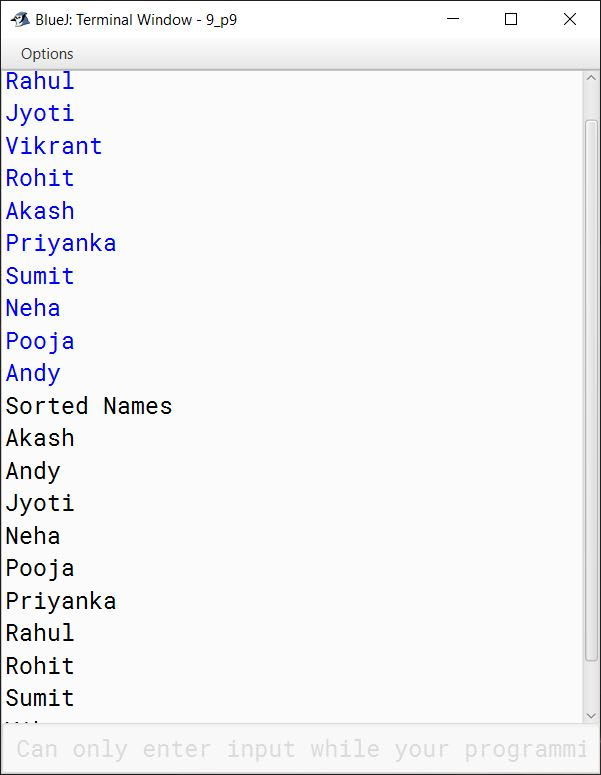# Solved 2017 Question Paper ICSE Class 10 Computer Applications

## Section A

#### Question 1

(a) What is inheritance?

Inheritance is the mechanism by which a class acquires the properties and methods of another class.

(b) Name the operators listed below:

1. <
2. ++
3. &&
4. ? :

1. Less than operator. (It is a relational operator)
2. Increment operator. (It is an arithmetic operator)
3. Logical AND operator. (It is a logical operator)
4. Ternary operator. (It is a conditional operator)

(c) State the number of bytes occupied by char and int data types.

char occupies 2 bytes and int occupies 4 bytes.

(d) Write one difference between / and % operator.

/ operator computes the quotient whereas % operator computes the remainder.

(e) String x[] = {"SAMSUNG", "NOKIA", "SONY", "MICROMAX", "BLACKBERRY"};

Give the output of the following statements:

1. System.out.println(x);
2. System.out.println(x.length());

1. The output of `System.out.println(x);` is NOKIA. `x` gives the second element of the array x which is "NOKIA"

2. The output of `System.out.println(x.length());` is 8. `x.length()` gives the number of characters in the fourth element of the array x which is MICROMAX.

#### Question 2

(a) Name the following:

1. A keyword used to call a package in the program.
2. Any one reference data type.

1. import
2. Array

(b) What are the two ways of invoking functions?

Call by value and call by reference.

(c) State the data type and value of res after the following is executed:

```char ch='t';
res= Character.toUpperCase(ch);```

Data type of res is char and its value is T.

(d) Give the output of the following program segment and also mention the number of times the loop is executed:

```int a,b;
for (a = 6, b = 4; a <= 24; a = a + 6)
{
if (a%b == 0)
break;
}
System.out.println(a);```

Output of the above code is 12 and loop executes 2 times.

##### Explanation

This dry run explains the working of the loop.

abRemarks
641st Iteration
1242nd Iteration

In 2nd iteration, as a%b becomes 0 so break statement is executed and the loop exits. Program control comes to the println statement which prints the output as current value of a which is 12.

(e) Write the output:

```char ch = 'F';
int m = ch;
m=m+5;
System.out.println(m + " " + ch);```

Output of the above code is:

``````75 F
``````
##### Explanation

The statement `int m = ch;` assigns the ASCII value of F (which is 70) to variable m. Adding 5 to m makes it 75. In the println statement, the current value of m which is 75 is printed followed by space and then value of ch which is F.

#### Question 3

(a) Write a Java expression for the following:
ax5 + bx3 + c

a * Math.pow(x, 5) + b * Math.pow(x, 3) + c

(b) What is the value of x1 if x=5?
x1= ++x – x++ + --x

x1 = ++x – x++ + --x
⇒ x1 = 6 - 6 + 6
⇒ x1 = 0 + 6
⇒ x1 = 6

(c) Why is an object called an instance of a class?

A class can create objects of itself with different characteristics and common behaviour. So, we can say that an Object represents a specific state of the class. For these reasons, an Object is called an Instance of a Class.

(d) Convert following do-while loop into for loop.

```int i = 1;
int d = 5;
do {
d=d*2;
System.out.println(d);
i++ ; } while ( i<=5);```

```int i = 1;
int d = 5;
for (i = 1; i <= 5; i++) {
d=d*2;
System.out.println(d);
}```

(e) Differentiate between constructor and function.

ConstructorFunction
Constructor is a block of code that initializes a newly created object.Function is a group of statements that can be called at any point in the program using its name to perform a specific task.
Constructor has the same name as class name.Function should have a different name than class name.
Constructor has no return type not even void.Function requires a valid return type.

(f) Write the output for the following:

```String s="Today is Test";
System.out.println(s.indexOf('T'));
System.out.println(s.substring(0,7) + " " +"Holiday");```

Output of the above code is:

``````0
Today i Holiday
``````
##### Explanation

As the first T is present at index 0 in string s so `s.indexOf('T')` returns 0. s.substring(0,7) extracts the characters from index 0 to index 6 of string s. So its result is Today i. After that println statement prints Today i followed by a space and Holiday.

(g) What are the values stored in variables r1 and r2:

1. double r1 = Math.abs(Math.min(-2.83, -5.83));
2. double r2 = Math.sqrt(Math.floor(16.3));

1. r1 has 5.83
2. r2 has 4.0
##### Explanation
1. Math.min(-2.83, -5.83) returns -5.83 as -5.83 is less than -2.83. (Note that these are negative numbers). Math.abs(-5.83) returns 5.83.
2. Math.floor(16.3) returns 16.0. Math.sqrt(16.0) gives square root of 16.0 which is 4.0.

(h) Give the output of the following code:

```String A ="26", B="100";
String D=A+B+"200";
int x= Integer.parseInt(A);
int y = Integer.parseInt(B);
int d = x+y;
System.out.println("Result 1 = " + D);
System.out.println("Result 2 = " + d);```

Output of the above code is:

``````Result 1 = 26100200
Result 2 = 126
``````
##### Explanation
1. As A and B are strings so `String D=A+B+"200";` joins A, B and "200" storing the string "26100200" in D.
2. Integer.parseInt() converts strings A and B into their respective numeric values. Thus, x becomes 26 and y becomes 100.
3. As Java is case-sensitive so D and d are treated as two different variables.
4. Sum of x and y is assigned to d. Thus, d gets a value of 126.

(i) Analyze the given program segment and answer the following questions:

```for(int i=3;i<=4;i++ ) {
for(int j=2;j<i;j++ ) {
System.out.print("" ); }
System.out.println("WIN" ); }```
1. How many times does the inner loop execute?
2. Write the output of the program segment.

1. Inner loop executes 3 times.
(For 1st iteration of outer loop, inner loop executes once. For 2nd iteration of outer loop, inner loop executes two times.)

2. Output:
WIN
WIN

(j) What is the difference between the Scanner class functions next() and nextLine()?

next()nextLine()
It reads the input only till space so it can read only a single word.It reads the input till the end of line so it can read a full sentence including spaces.
It places the cursor in the same line after reading the input.It places the cursor in the next line after reading the input.

## Section B

#### Question 4

Define a class ElectricBill with the following specifications:

class : ElectricBill

Instance variables / data member:
String n — to store the name of the customer
int units — to store the number of units consumed
double bill — to store the amount to be paid

Member methods:
void accept( ) — to accept the name of the customer and number of units consumed
void calculate( ) — to calculate the bill as per the following tariff:

Number of unitsRate per unit
First 100 unitsRs.2.00
Next 200 unitsRs.3.00
Above 300 unitsRs.5.00

A surcharge of 2.5% charged if the number of units consumed is above 300 units.

void print( ) — To print the details as follows:
Name of the customer: ………………………
Number of units consumed: ………………………
Bill amount: ………………………

Write a main method to create an object of the class and call the above member methods.

```import java.util.Scanner;

public class ElectricBill
{
private String n;
private int units;
private double bill;

public void accept() {
Scanner in = new Scanner(System.in);
System.out.print("Enter customer name: ");
n = in.nextLine();
System.out.print("Enter units consumed: ");
units = in.nextInt();
}

public void calculate() {
if (units <= 100)
bill = units * 2;
else if (units <= 300)
bill = 200 + (units - 100) * 3;
else {
double amt = 200 + 600 + (units - 300) * 5;
double surcharge = (amt * 2.5) / 100.0;
bill = amt + surcharge;
}
}

public void print() {
System.out.println("Name of the customer\t\t: " + n);
System.out.println("Number of units consumed\t: " + units);
System.out.println("Bill amount\t\t\t: " + bill);
}

public static void main(String args[]) {
ElectricBill obj = new ElectricBill();
obj.accept();
obj.calculate();
obj.print();
}
}```
##### Output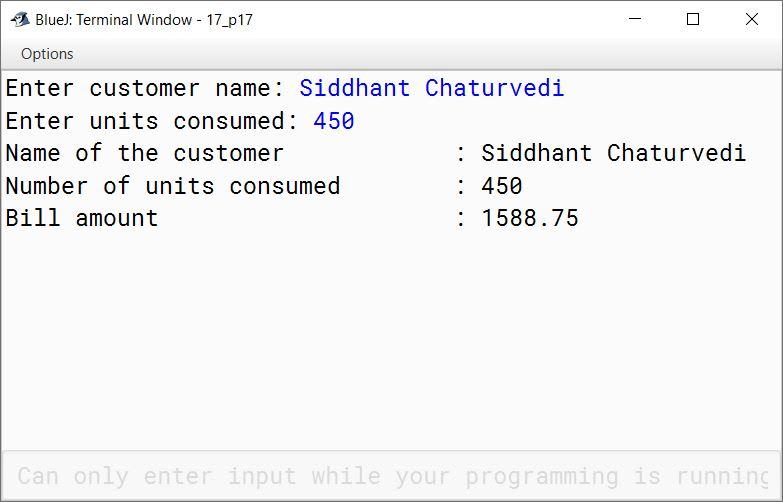#### Question 5

Write a program to accept a number and check and display whether it is a spy number or not. (A number is spy if the sum of its digits equals the product of its digits.)

Example: consider the number 1124.
Sum of the digits = 1 + 1 + 2 + 4 = 8
Product of the digits = 1 x 1 x 2 x 4 = 8

```import java.util.Scanner;

public class KboatSpyNumber
{
public static void main(String args[]) {

Scanner in = new Scanner(System.in);

System.out.print("Enter Number: ");
int num = in.nextInt();

int digit, sum = 0;
int orgNum = num;
int prod = 1;

while (num > 0) {
digit = num % 10;

sum += digit;
prod *= digit;
num /= 10;
}

if (sum == prod)
System.out.println(orgNum + " is Spy Number");
else
System.out.println(orgNum + " is not Spy Number");

}
}```
##### Output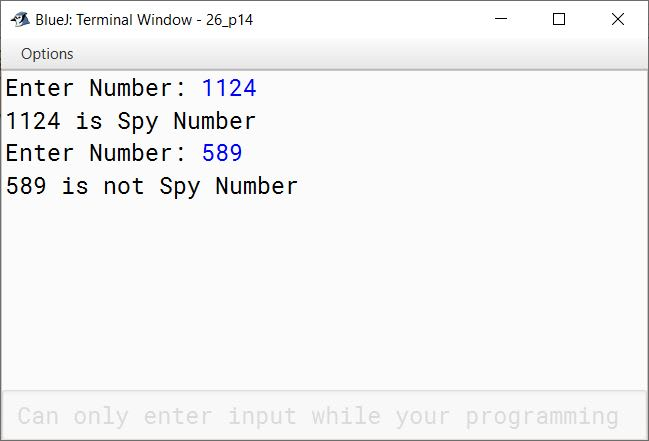#### Question 6

Using switch statement, write a menu driven program for the following:

1. To find and display the sum of the series given below:
S = x1 - x2 + x3 - x4 + x5 .......... - x20
(where x = 2)
2. To display the following series:
1 11 111 1111 11111

For an incorrect option, an appropriate error message should be displayed.

```import java.util.Scanner;

{
public static void main(String args[]) {
Scanner in = new Scanner(System.in);
System.out.println("1. Sum of the series");
System.out.println("2. Display series");
int choice = in.nextInt();

switch (choice) {
case 1:
int sum = 0;
for (int i = 1; i <= 20; i++) {
int x = 2;
int term = (int)Math.pow(x, i);
if (i % 2 == 0)
sum -= term;
else
sum += term;
}
System.out.println("Sum=" + sum);
break;

case 2:
int term = 1;
for (int i = 1; i <= 5; i++) {
System.out.print(term + " ");
term = term * 10 + 1;
}
break;

default:
System.out.println("Incorrect Choice");
break;
}
}
}```
##### Output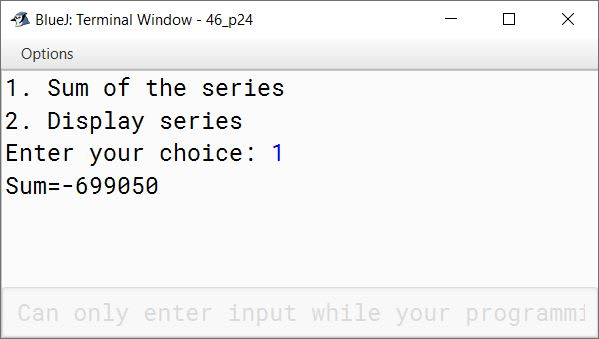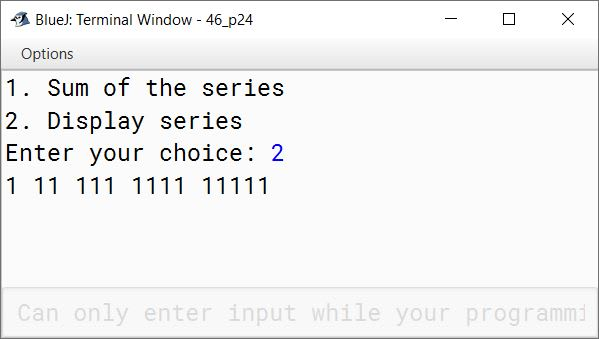#### Question 7

Write a program to input integer elements into an array of size 20 and perform the following operations:

1. Display largest number from the array.
2. Display smallest number from the array.
3. Display sum of all the elements of the array

```import java.util.Scanner;

public class KboatSDAMinMaxSum
{
public static void main(String args[]) {
Scanner in = new Scanner(System.in);
int arr[] = new int;
System.out.println("Enter 20 numbers:");
for (int i = 0; i < 20; i++) {
arr[i] = in.nextInt();
}
int min = arr, max = arr, sum = 0;
for (int i = 0; i < arr.length; i++) {
if (arr[i] < min)
min = arr[i];

if (arr[i] > max)
max = arr[i];

sum += arr[i];
}

System.out.println("Largest Number = " + max);
System.out.println("Smallest Number = " + min);
System.out.println("Sum = " + sum);
}
}```
##### Output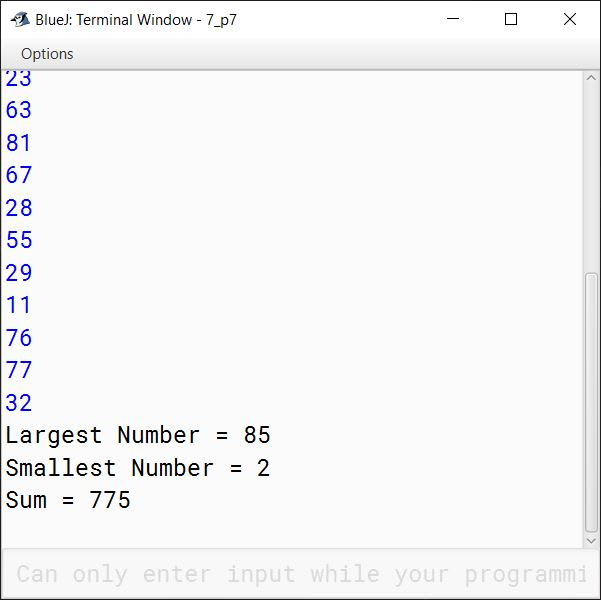#### Question 8

Design a class to overload a function check( ) as follows:

1. void check (String str , char ch ) — to find and print the frequency of a character in a string.
Example:
Input:
str = "success"
ch = 's'
Output:
number of s present is = 3

2. void check(String s1) — to display only vowels from string s1, after converting it to lower case.
Example:
Input:
s1 ="computer"
Output : o u e

```public class KboatOverload
{
void check (String str , char ch ) {
int count = 0;
int len = str.length();
for (int i = 0; i < str.length(); i++) {
char c = str.charAt(i);
if (ch == c) {
count++;
}
}
System.out.println("Frequency of " + ch + " = " + count);
}

void check(String s1) {
String s2 = s1.toLowerCase();
int len = s2.length();
System.out.println("Vowels:");
for (int i = 0; i < len; i++) {
char ch = s2.charAt(i);
if (ch == 'a' ||
ch == 'e' ||
ch == 'i' ||
ch == 'o' ||
ch == 'u')
System.out.print(ch + " ");
}
}
}```
##### Output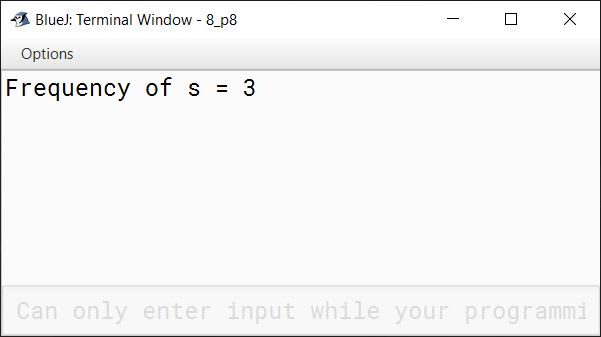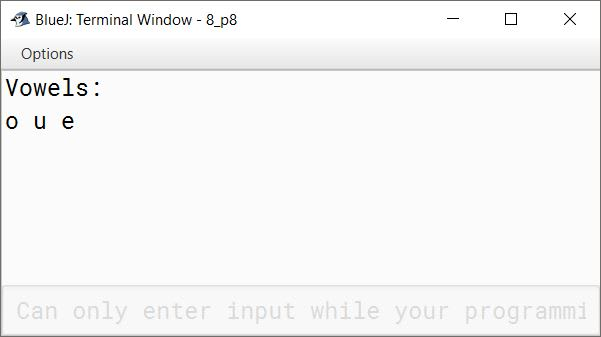#### Question 9

Write a program to input forty words in an array. Arrange these words in descending order of alphabets, using selection sort technique. Print the sorted array.

```import java.util.Scanner;

public class KboatSelectionSort
{
public static void main(String args[]) {
Scanner in = new Scanner(System.in);
String a[] = new String;
int n = a.length;

System.out.println("Enter 40 Names: ");
for (int i = 0; i < n; i++) {
a[i] = in.nextLine();
}

for (int i = 0; i < n - 1; i++) {
int idx = i;
for (int j = i + 1; j < n; j++) {
if (a[j].compareToIgnoreCase(a[idx]) < 0) {
idx = j;
}
}
String t = a[idx];
a[idx] = a[i];
a[i] = t;
}

System.out.println("Sorted Names");
for (int i = 0; i < n; i++) {
System.out.println(a[i]);
}
}
}```
##### Output